## 19.3.3 The styling section

The styling section can contain any of the following settings in any order:

 arrow_headAngle Possible values: Any floating-point number. Sets the angle, in degrees, at which the two sides of arrow heads meet at its point. arrow_headSize Possible values: Any floating-point number. Sets the size of all arrow heads. A value of 1.0 corresponds to Pyxplot’s default size. arrow_headBackIndent Possible values: Any floating-point number. Sets the size of the indentation in the back of arrow heads. The default size is 0.2. Sensible values lie in the range 0 (no indentation) to 1 (the indentation extends the whole length of the arrow head). Less sensible values may be used by the aesthetically adventurous. axes_lineWidth Possible values: Any floating-point number. Sets the line width used to draw graph axes. axes_majTickLen Possible values: Any floating-point number. Sets the length of major axis ticks. A value of 1.0 corresponds to Pyxplot’s default length of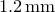; other values differ from this multiplicatively. axes_minTickLen Possible values: Any floating-point number. Sets the length of minor axis ticks. A value of 1.0 corresponds to Pyxplot’s default length of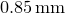; other values differ from this multiplicatively. axes_separation Possible values: Any floating-point number. Sets the separation between parallel axes on graphs, less the width of any text labels associated with the axes. A value of 1.0 corresponds to Pyxplot’s default spacing of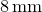; other values differ from this multiplicatively. axes_textGap Possible values: Any floating-point number. Sets the separation between axes and the text labels which are associated with them. A value of 1.0 corresponds to Pyxplot’s default spacing of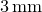; other values differ from this multiplicatively. colorScale_margin Possible values: Any floating-point number. Sets the separation left between the axes of plots drawn using the colormap plot style, and of the color scales drawn alongside them. A value of 1.0 corresponds to Pyxplot’s default spacing; other values differ from this multiplicatively. colorScale_width Possible values: Any floating-point number. Sets the width of the color scale bars drawn alonside plots drawn using the colormap plot style. A value of 1.0 corresponds to Pyxplot’s width; other values differ from this multiplicatively. grid_majLineWidth Possible values: Any floating-point number. Sets the line width used to draw major gridlines (default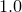). grid_minLineWidth Possible values: Any floating-point number. Sets the line width used to draw minor gridlines (default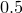). baseline_lineWidth Possible values: Any floating-point number. Sets the PostScript line width which corresponds to a linewidth of 1.0. A value of 1.0 corresponds to Pyxplot’s default line width of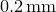; other values differ from this multiplicatively. baseline_pointSize Possible values: Any floating-point number. Sets the baseline point size which corresponds to a pointsize of 1.0. A value of 1.0 corresponds to Pyxplot’s default; other values differ from this multiplicatively.